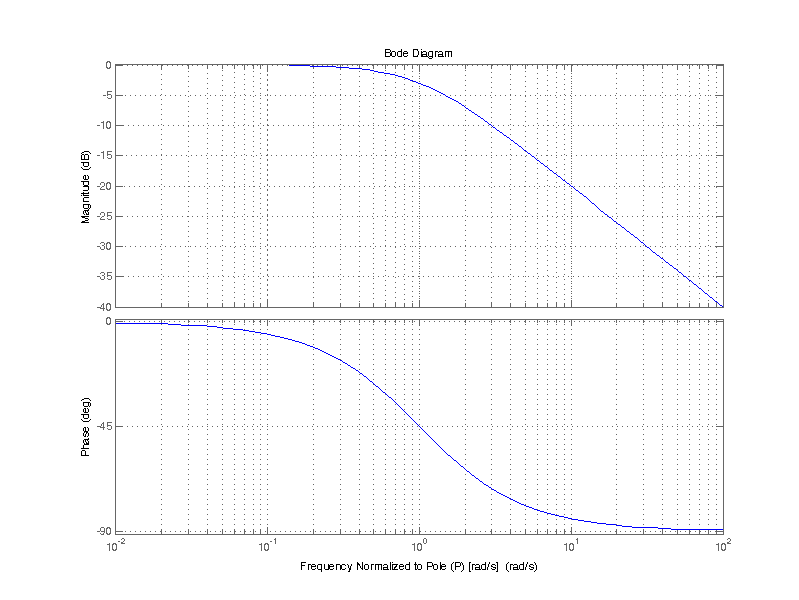# Single Pole Transfer Function

A single pole transfer function has the general from of
$H(s) = \dfrac{1}{1 + \frac{s}{p}}$

Since H(s) has no roots in the numerator, the transfer function H(s) has no zeros. H(s) does have one root in the denominator, at

$s = -p$

H(s) is said to have one pole at -p in the left-hand plane. As will be seen in the following sections, there are some important attributes for the magnitude and phase of a transfer function which can be generalized around the pole location.

From complex number theory the magnitude-squared of a function can be related as

$\left| H(s) \right|^2 = H(s) \cdot H^*(s)$

For $$s = j\omega$$

where the astrix is the complex conjugate of H
$H^*(j\omega) = H(-j\omega)$

\begin{align*}
\left| H(j\omega) \right| ^2 &= H(j\omega)H(-j\omega) \\
&= \left( \dfrac{1}{1 + \frac{j\omega}{p}} \right)\left( \dfrac{1}{1 + \frac{-j\omega}{p}} \right) \\
\left| H(j\omega) \right| ^2 &= \dfrac{1}{1 + \frac{\omega^2}{p^2} }
\end{align*}

When $$\omega = p$$, $$\left| H(j\omega) \right| ^2 = 1/2$$

Finally the magnitude of H(jw) then becomes
$|H(j\omega)| = \dfrac{1}{\sqrt{1 + \frac{\omega^2}{p^2} }}$

The phase response of H(jw) is related by the real and imaginary components of H(jw) as follows
$\angle H(j\omega) = arg\left( H(j\omega )\right) = tan^{-1}\left( \dfrac{\mathbb{I}\left( H(j\omega) \right)}{\mathbb{R}\left( H(j\omega) \right)} \right)$

Normalizing H(jw) into the form $$a + jb$$ is as follows
\begin{align*}
H(j\omega) &= \dfrac{1}{1 + \frac{j\omega}{p}}\\
&= \left(\dfrac{1}{1 + \frac{j\omega}{p}}\right) \left( \dfrac{1 – \frac{j\omega}{p}}{1 – \frac{j\omega}{p}} \right)\\
H(j\omega)&= \dfrac{1}{1 + \frac{\omega^2}{p^2}} – j\left( \dfrac{\omega/p}{1 + \frac{\omega^2}{p^2}} \right)
\end{align*}

The real component is
$\mathbb{R}\left( H(j\omega) \right) = \dfrac{1}{1 + \frac{\omega^2}{p^2}}$

The imaginary component is
$\mathbb{I}\left( H(j\omega) \right) = \dfrac{-\omega/p}{1 + \frac{\omega^2}{p^2}}$

The phase of H(jw) is then
$\angle H(j\omega) = tan^{-1} \left( \dfrac{\omega}{p} \right)$

It’s worth illustrating that the reason the phase sweeps near the pole P is due to the fact that the real component declines at $$1/\omega^2$$, where as the imaginary component declines slower at $$1/\omega$$.

\begin{align*}
| H(j\omega)| &= \sqrt{\left(\mathbb{R}\left( H(j\omega) \right)\right)^2 + \left(\mathbb{I}\left( H(j\omega) \right)\right)^2 } \\
&= \sqrt{\left( \dfrac{1}{1 + \frac{\omega^2}{p^2}} \right)^2+\left( \dfrac{\omega/p}{1 + \frac{\omega^2}{p^2}}\right)^2} \\
&= \sqrt{\dfrac{1 + \frac{\omega^2}{p^2}}{\left(1 + \frac{\omega^2}{p^2} \right)^2}} \\
| H(j\omega)| &=\dfrac{1}{\sqrt{1 + \frac{\omega^2}{p^2} }}
\end{align*}

The magnitude of H computed from the real and imaginary components is equivalent to the square root of the magnitude-squared relation.
$| H(j\omega)| = \sqrt{H(j\omega)H(-j\omega)}$

The figure below is a bode plot of a the single pole transfer function H(s). Frequency $$\omega$$ is normalized to pole P, i.e. the ratio $$\omega/P$$ is plotted on the x-axis. The top half of the plot depicts the magnitude, the bottom half the phase. Magnitude is plotted on a 20log10 dB scale.There are a few important attributes for magnitude and phase of H(jw) near the pole P. The magnitude is left unperturbed from its DC value, in this case unity or 0 dB, up until omega equals P. When $$\omega = P$$, the magnitude “breaks” at -20 dB/decade, and continue indefinitely. The phase of H(jw) begin to decline at 0.1 P up until 10 P, at a rate of -45 deg/decade.

The table below provides the exact evaluation of H(jw) at some normalized frequencies before and after the Pole P.

Frequency Response of H(jw)
Normalized Frequency (w/p) Real(H(jw)) Imag(H(jw)) Magnitude (dB) Phase (deg)
0.01 0.9999 0.0100 -0.0004 -0.6
0.1 0.9901 0.0990 -0.0432 -5.7
1 0.5000 0.5000 -3.0103 -45.0
10 0.0099 0.0990 -20.0432 -84.3
100 0.0001 0.0100 -40.0004 -89.4

A single pole transfer function may also be described as a low-pass filter. Where the passband (the signals of interest) is from DC up until when $$\omega_c=2\pi f_c = P$$. Beyond the corner frequency signals are rejected (attenuated). However in the case of a single pole filter, a signal at frequency of 2*fc is only attenuated by 0.45 or -7 dB. It isn’t until frequencies of 10x or 100x fc that the attenuation becomes -20 dB or -40 dB respectively.

When attenuation of much greater than a few dB is desired just after the corner frequency (i.e a sharp roll-off) a higher order filter is required.

## 1 thought on “Single Pole Transfer Function”

1.Michael Segev says:

Excellent explanation!

This site uses Akismet to reduce spam. Learn how your comment data is processed.